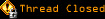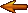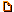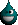# Topic awaiting preservation: Orbital Motion Algorithm (Page 1 of 1)WarMage

From: Rochester, New York, USA
Insane since: May 2000posted 01-20-2004 20:02

I am working on getting some basic information down for a project I am working on which will involve modeling the solar system (in a very basic way). The hard part I am coming up against is that I can not find a simple algorithm to present me with <x,y,z> coordinates for the position of the center of a sphere rotation around a fixed point in my case <0,0,0>.

Lets for instance look at mercury:
distance from sun(km): 57,910,000 -> 579.1 units might be more useful
orbital period(days): 87.969 // note that either the period
orbital velocity(km/s): 47.88 // or velocity might be used
orbital inclination(degrees): 7.00

So I am thinking that given a starting point for mercurty <0,0,579.1> I would be able to use
r = 579.1
T = 87.969
i = 7.00
v = <0,0,579.1>

in a forumula to calculate its motion at an arbitrary intervals of say 1 period unit (1 day). I would also figure that this algorithm would be something that might be found rather often for those who regulary do 3d programming. However, it turns out that I can not find a simple algorithm that would define orbital motion, and I keep getting referenced to books on orbital mechanics which I am sure would give me far more information than I might need to solve this problem.

If you have any ideas, knowledge or links you could share with me I would appreciate it.

-Dan-

Rooster
Bipolar (III) Inmate

From: the uterus
Insane since: Nov 2002posted 01-20-2004 20:39
quote:
The hard part I am coming up against is that I can not find a simple algorithm to present me with <x,y,z> coordinates for the position of the center of a sphere rotation around a fixed point in my case <0,0,0>.

Have you tried researching the spherical coordinate system?

WarMage

From: Rochester, New York, USA
Insane since: May 2000posted 01-20-2004 21:06

No, I actually didn't know it even existed.

Let me see...

<rho,phi,theta>
rho = distance from origin
phi = latitude
theta = longitude

x = (rho)*sin(phi)*cos(theta)
y = (rho)*sin(phi)*sin(theta)
z = (rho)*cos(phi)

With this I easily can reprisent the distance as rho, my phi would be calculated based on a moving angle of inclination while my theta would be a measure of the period.

I would further state that I can leave out the angle of inclination and set my phi to 0 degrees, saying that all the planets are aligned (more because I can not think up the math to make this change).

My theta would still be veriable. But the function works out simply as:
theta = 360 degrees / period of orbit (I say this as 360 degrees is the total number of degrees in the circle)

This would bring me to the end result of

x = (distance from sun) * sin(0) * cos(360/period)
y = (distance from sun) * sin(0) * sin(360/period)
z = (distance from sun) * cos(0)

At this point I have myself rather confused, becuase sin(0) = 0 which really throws the whole equation off. Am I miss understanding the usage of phi?

-Dan-

[This message has been edited by WarMage (edited 01-20-2004).]

Veneficuz
Paranoid (IV) Inmate

From: A graveyard of dreams
Insane since: Mar 2001posted 01-20-2004 21:31

The usage of phi is a little bit different. 0 phi points straight up along the z-axis. That is why a phi of 0 gives you x=0, y=0. So to have the planet rotate in the xy-plane you've got to use phi=90 degrees. So sin(90)=1, cos(90)=0 and that gives you the following formulas:
x = (distance from sun) * cos(360/period)
y = (distance from sun) * sin(360/period)
z = 0
Which seem more correct

_________________________
"There are 10 kinds of people; those who know binary, those who don't and those who start counting at zero"
- the Golden Ratio -

WarMage

From: Rochester, New York, USA
Insane since: May 2000posted 01-20-2004 21:40

Excellent, thanks a lot!

-Dan-

Halma
Nervous Wreck (II) Inmate

From:
Insane since: Jan 2004posted 01-29-2004 13:27

However, please note that in your formulations, the angular velocity of the orbiting planet is constant. (You are assuming that it moves exactly (360/period) degrees every day)

This is not accurate.

An object orbiting a larger object (eg a planet orbiting the sun) will assume an elliptical path in which the large object (eg sun) is one of the foci.. (With a circle being a rare special-case of an ellipse.) The linear velocity of the planet is highest when it is closest to the sun.. and least when it is farthest from the sun. This difference of velocities is amplified when you're working with angular velocities, because as the planet gets closer to the sun, the angular velocity/linear velocity ratio also increases! (When you're close to the sun, 1 meter gives a much larger angle than 1 meter at a location far from the sun)

The most accurate way to calculate it is to use the laws of gravitation+inertia to derive the exact velocities and therefore coordinates..

But, of course, using constant angular velocity will probably look good enough for a not-too-scientific animation..(I know it would fool me!)